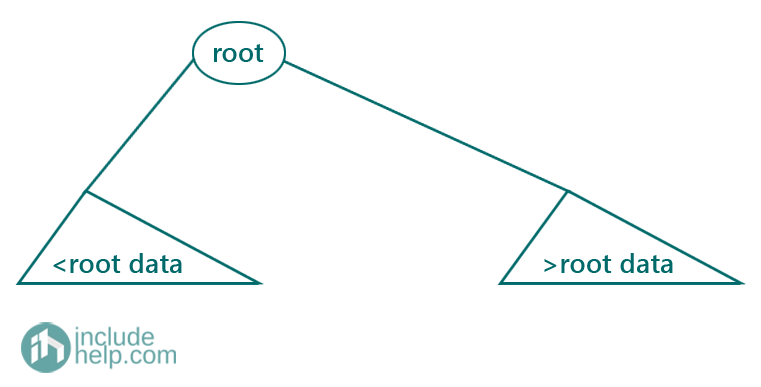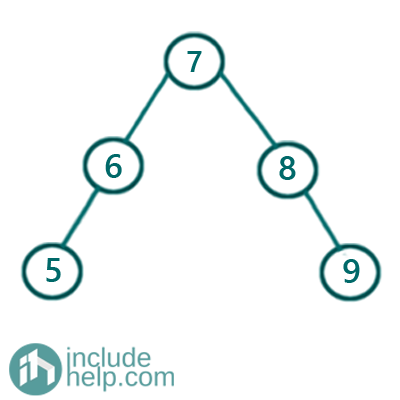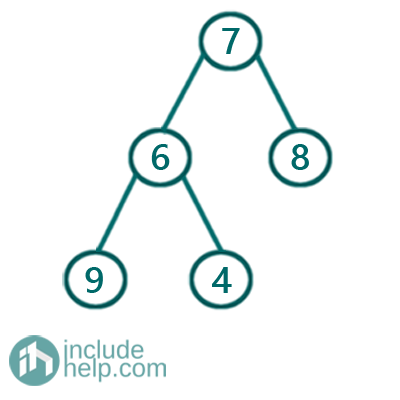# Introduction to Binary Search Tree (BST)

In this article, we are going to see what is binary search tree and why do we require it and what are properties of a binary tree?
Submitted by Radib Kar, on September 16, 2020

We already know that Binary search Tree is a special kind of Binary Tree which is a very efficient data structure for searching. In Normal binary trees, the parent has no relationship with the child in terms of values, or say there is no restriction on nodes data. That's why while searching an element in a normal Binary Tree, we need to search the entire tree or say, the search is not at all guided resulting in the Time complexity of O(n).

There comes the need of a Binary Search Tree. A tree is a hierarchical data structure and we expect the time complexity of the ADT operations to be concerning the tree height, not concerning tree size. In the case of Binary search tree, there is a restriction in the node data population which results in a better searching time complexity, on average O(log N) or O(h) where h be the tree height.

## Binary Search Tree Property

1. All node values must be distinct
2. Parent value must be greater than the left child value and smaller than the right child value
3. Both the left and right subtree needs to be Binary search tree as well
4. All the node in the left subtree of the root has less key/node value than the root
5. All the node in the right subtree of the root has greater key/node value than the rootExamples:

1. BST:
Below is the example of a BST as it maintains all the properties.2. Not a BST:
Below is the example which is not a BST as the left subtree (rooted by 6) is not a BST here.ADT operation on Binary Search Trees:

1. Insert a Node (Insertion in a Binary Search Tree)
2. Delete a Node (Deletion in Binary Search Tree)
3. Search a Node
4. Find Minimum node value
5. Find Maximum node value

Few More Interesting Points/Properties

1. Inorder traversal of the binary search trees produces a sorted list since all node in the left subtree of the root is smaller than root and all nodes in the right subtree of the root are greater than the root.
2. Searching doesn't require to search the whole tree as we can search only in one subtree depending on search value. If the search value is greater than root value then search in the subtree only, if it's less then search in the left subtree only. That's why the search is more efficient and have a complexity of O(log N)
3. Though a BST is there to endure the searching efficiency, still it can result in a skew tree like below:The above tree is a right skew tree and each node has only the right child. So though it's a BST, searching will have complexity O(h) which is O(n) in this case.The above tree is a left skew tree and each node has only the left child. So though it's a BST, searching will have complexity O(h) which is O(n) in this case.

## Binary Search Tree Tutorial

Basic

Construction & Conversion

Checking & Searching

Languages: » C » C++ » C++ STL » Java » Data Structure » C#.Net » Android » Kotlin » SQL
Web Technologies: » PHP » Python » JavaScript » CSS » Ajax » Node.js » Web programming/HTML
Solved programs: » C » C++ » DS » Java » C#
Aptitude que. & ans.: » C » C++ » Java » DBMS
Interview que. & ans.: » C » Embedded C » Java » SEO » HR
CS Subjects: » CS Basics » O.S. » Networks » DBMS » Embedded Systems » Cloud Computing
» Machine learning » CS Organizations » Linux » DOS
More: » Articles » Puzzles » News/Updates